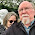## Sunday, November 10, 2013

### Factoring ... which method works best?

In a week, we will start our unit on solving quadratics.  We are in the midst of a short unit on the characteristics of quadratics where we talk about the vertex, axis of symmetry, the intercepts, the standard form, the vertex form, etc.  Students are adept at analyzing a graph of a parabola and interpreting the meaning of specific values in problem situations.

We have not yet made the connection between the x-intercepts, zeros, and solutions to quadratic equations.  I will start there as an introduction to the unit on solving.

And then we will review factoring.  Right now, the plan is to use at least 3 methods in factoring ...

In all of these methods we use the "X" puzzle to help students organize their factors.

Last year I refused to teach my Algebra 1 students the Kick It method.  It seemed so contrived at the time.  This year, I think I want to teach students what some call the AC method.  I read about it here.  The Kick It method and the AC method are very similar.  I don't believe it will be come the method of choice, but I will at least demonstrate it.  Factoring by grouping makes so much more sense to me.

Because of the way our curriculum department schedules units, we will have only one class period to review factoring!  It will be tight for sure.

As class practice I'll either use a circuit or play the spoons game!

If I use a circuit (a loop) ... students will work in pairs to factor problems posted around the room.  The solution set to one equation will be found on another poster ... they work that second problem ... look for the solution set ... and so on.  If they work the problems correctly, the loop closes.  I love self-checking practice.

If we play the spoons game, students will work in teams to factor equations.  Answer cards will be on the table - one less card than the number of teams.  I know my students would like this game!

After students have had practice with multiple methods of factoring, they will get to choose the method they prefer in the rest of the unit.

How do you teach factoring?

#### 3 comments:

1.I cannot get your link to work (the I read about it here link) but I suspect that I know and use what you call the AC method. I tell my students that quadratics of the form ax^2 + bx + c yield to this technique
1) Find the product ac
2) Find factors of ac that add to b - let's call them p and q
3) Rewrite ax^2 + bx + c as ax^2 + px + qx + c
4) Since p and q are factors of ac we know for sure that ax^2 + px has a common factor - let's call it rx. The remaining factor is of special interest.
5) Since p and q are factors of ac we know that qx + c will have a common factor - let's call is sx. The remaining factor when we pull out sx is exactly the same as the remaining factor from step 4. We know have decomposed ax^2 + bx + c

The reason I especially like this technique is that it plays into my disdain for FOIL-ing and shows how this process is simply the opposite of distributing to multiply binomials.
Hope this made sense!

1.The method you describe is what we call factoring by grouping. Do you teach any other method in your classes?

2.Not anymore. I used to place value on just sort of sifting through options in your head - but I feel that a systematic way (especially if it reinforces something else that's important) is a better approach.